Reach Us+44-1904-929220
The Mathematical Treatment for Air Pollutant Diffusion Using Laplace Adomain Decomposition Method with Ground Surface | OMICS International
Journal of Civil & Environmental Engineering
All submissions of the EM system will be redirected to Online Manuscript Submission System. Authors are requested to submit articles directly to Online Manuscript Submission System of respective journal.

# The Mathematical Treatment for Air Pollutant Diffusion Using Laplace Adomain Decomposition Method with Ground Surface

 Esmail S* and Mayhoub AB Department of Mathematics and Theoretical Physics, Atomic Energy Authority, Cairo, Egypt *Corresponding Author : Samia Esmail Department of Mathematics and Theoretical Physics Atomic Energy Authority, Cairo, Egypt Tel:+20 2 22730263 E-mail: [email protected] Received August 18, 2015; Accepted January 25, 2016; Published January 28,2016 Citation:Esmail S, Mayhoub AB (2016) The Mathematical Treatment for Air Pollutant Diffusion Using Laplace Adomain Decomposition Method with Ground Surface. J Civil Environ Eng 6:212. doi:10.4172/2165-784X.1000212 Copyright: © 2016 Esmail S, et al. This is an open-access article distributed under the terms of the Creative Commons Attribution License, which permits unrestricted use, distribution, and reproduction in any medium, provided the original author and source are credited.

Visit for more related articles at Journal of Civil & Environmental Engineering

#### Abstract

The method of Laplace Adomain Decomposition has been used to obtain a semi-analytical solution of the threedimensional steady state advection diffusion equation for dispersion of air pollutant from a point source. The present treatment takes into account a realistic boundary condition which considers the ground surface as an absorberreflector surface for the pollutant, simultaneously. This physical consideration is achieved by assuming that the vertical eddy diffusivity coefficient should be non-zero at the ground surface for vertical diffusion to be possible. The wind prevailing speed is parameterized in terms of vertical height using the power law profile. An upper boundary condition assuming capping inversion is considered which means that pollutant is subjected to a boundary Condition of zero flux. The present model calculations are compared with the available data of the atmospheric dispersion experiments that were carried out in the Copenhagen area (Denmark) and the semi-empirical model for Gaussian plume model with the same input data. In both comparison tasks, the results are reasonably good which indicates that the present treatment performs well as a simple analytical dispersion model.

 Keywords Air pollutant; Decomposition method; Ground surface; Mathematical treatment Introduction In the last few decades, special attention has been devoted to the task of predicting analytical solutions for the atmospheric dispersion of pollutants in the planetary boundary layer (PBL). Presently, analytical solutions of the advection diffusion equation are usually obtained for steady state conditions with assuming specific formulae for both eddy diffusivity (k) and (u). They are assumed as constants through the PBL or follow a power law . Despite that k profile is not physically realistic; the solutions of the dispersion equation are widely used for regulatory application, because they can give generally, a qualitative concepts and reasonable description of the pollutant dispersion within the PBL. In the frame work of analytical solutions for the sake of illustration, we draw the attention to the work of Sharan et al. , Moreira et al.  and Wortmann et al. . For more details, refer to the work of Vilhena et al.  and Degrazia et al. . Different mathematical treatments have been adopted in the above mentioned literatures such as, Laplace transform technique with numerical inversion. A step forward is taken by Wortmann et al.  by application of the generalized integral Laplace transform technique (GILTT) to solve the two-dimensional advection diffusion equation. In general, dispersion modeling depends principally on parameterization of the eddy diffusivity in terms of the physical parameter of the dispersion process. Also it depends on the suitable realistic boundary conditions assumed at the ground level and the top of the PBL which work reasonable well during most meteorological regimes. Various parameterizations for the diffusivity have been made in the literature to explain dispersion process. As examples of these researches, Sharan et al. expressed the diffusivity coefficients ky and kz in y and z directions in terms of the standard deviations ¾y and ¾z, the standard deviations of the crosswind and vertical concentration distribution respectively. Moreira et al.  put forward a new parameterization of the diffusivity coefficient kz by assuming that the height of the PBL can be discretized in N sub-intervals in such a manner that inside each interval kz take an average constant value. In this work, we will present the solution for the 3-dimensional advection diffusion equation in non-stationary condition applying Laplace Adomain de-composition technique. Laplace Adomain decomposition is used for solving our problem; this method is a numerical algorithm to solve linear and non-linear partial differential equations and ordinary differential equations. Khuri is the first one who used this method. Agadjanov applied this method for the solution of Duffing equation, Sujit Handibag and . Karande solved linear and non-linear heat equation using this method. This numerical technique basically illustrates how Laplace transform may be used to approximate the solutions by manipulating the de- composition method which was first introduced by Adomain. Also, the optimal homotopy analysis method (HAM) and Differential Transform Method (DTM) are applied to study the magneto-hemodynamic laminar viscous flow of a conducting physiological fluid in a semi-porous channel under a transverse magnetic field , Erfani and Rashidi use the modified differential transform method to solve an off-centered stagnation flow toward a rotating disc  The paper is organized in 3 sections. Section 1 is an introduction. Section 2 presents the mathematical formulation of the solution of the advection-diffusion equation together with a brief exhibition to Gaussian plume model (the most widespread dispersion model). Section 3 presents the application of this solution and their comparison with both experimental data which were collected from the northern part of Copenhagen  and the most widespread dispersion model, namely Gaussian plume model output. Mathematical Treatment The advection diffusion equation of an air pollutant in the atmosphere is essentially a statement of conservation of the suspended material. The concentration 2 due to turbulent fluxes are assumed to be proportional to the mean concentration gradient which is known as Fick-theory. This assumption, combined with the continuity equation, leads to the steady-state advection diffusion equation Blackadar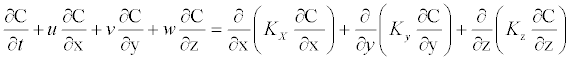(1) Where C(x, y, z) denotes the concentration, kx; ky and kz are the Cartesian components of eddy diffusivity and u; v and w are the Cartesian components of wind speed. In order to solve the equation (1) we included the following assumptions: (i) the pollutants are inert and have no additional sinks or sources downwind from the point source, (ii) the vertical w and lateral v components of the mean flow are assumed to be zero, kx is function of down distance (x) and the ground is considered as an absorber-reflector surface for the pollutant. This realistic boundary condition is achieved by expressing the diffusivity coefficient kz as a sum of two terms. The first one is of constant value which represents the value of kz at the ground level (z = 0), this is an essential requirement for the absorptive of the ground surface to the pollutants. The second term which is z-dependent (power law profile), represents the diffusivity coefficient at different heights above the ground (z > 0) and indicates the reflectivity of the ground surface to the pollutants, and (iii) the mean horizontal flow is incompressible and horizontally homogeneous (steady state). Then, we have where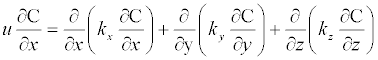(2)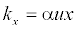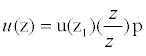(3)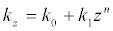(4) K0 is the eddy diffusivity at the ground, k1 is turbulent parameter, n and p are constants depend on the atmospheric stability classes. The mathematical description of the dispersion problem (2) is completed by the following boundary conditions: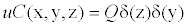at x = 0 (5)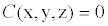at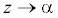(6)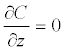at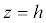;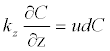at z=0 (7) Where vd is the deposition velocity which is constant forever element and Q is the emission rate. Taking integration for equation (2) with respect to y, we get: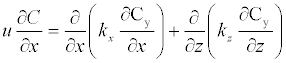(8) Where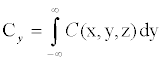is the cross-wind concentration. Applying Laplace transform (LT) for equation (8) with respect to x, we obtain: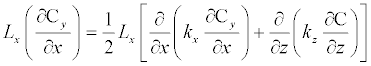(9)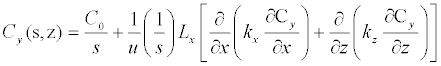(10) where C0 = Cy(0; z). By taking inverse Laplace transform for equation (10), we get: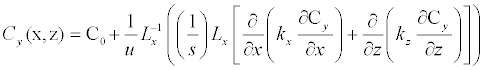(11) In Laplace decomposition method, the solution is assumed in infinite series form, Therefore we suppose that: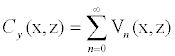(12) By substituting from Eqs. (12) and (6) into equation (11), we obtain: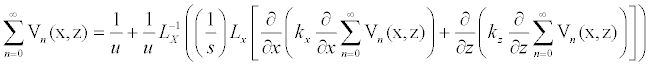(13) Comparing the two sides of the above equation, we get: u;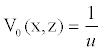,and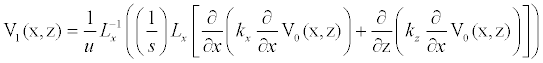(14) n general, the recursive relation is given by: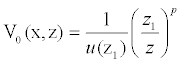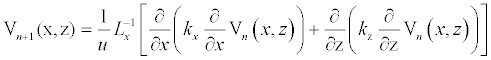(15) Using the above relation, n ≥ 0; we can find the components of V (x, z), namely V0, V1, V2,::::,Vn.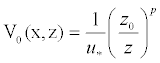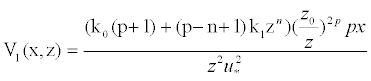(16) and so on. In this manner the rest of the components of the series (12) have been calculated using mathmod (mathematica programme). Substituting all these values in equation (12) in series form, we get the exact solution for general case: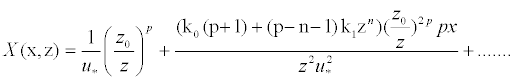(17) Where u* is the fraction velocity and z0 is the roughness length. Gaussian model The ground level Gaussian model of the concentration for an elevated source in the form :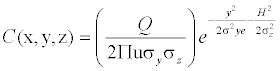(18) Describes the relationship between the ground level concentration C, source emission rate Q, effective height H, downwind speed u and the lateral and vertical dispersion coefficients ¾y and ¾z [11,12]. The dispersion coefficients determine how much the plume is dispersed and spread out in cross-wind direction y and in the vertical direction z as it is transported by the effect of the wind u in the x-direction and atmospheric thermal stability conditions (i.e. stability class). Integrating equation (18) w.r.to y, we obtain: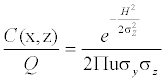(19) Where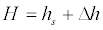,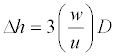Where is the exit velocity, hs is the stack height and D is the external diameter of the plume. Applications In order to examine the performance of the present model, crosswind integrated concentrations predicted by the model have been compared with the observed experimental data collected from the diffusion experiment carried out in the northern part of Copenhagen (Denmark)  and k1 is taken in the form :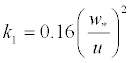,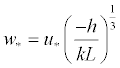Where * w is the convective velocity, L is the Monin Obukhov length and k = 0.4. Table 1 shows the comparison between our results using Laplace Adomain decomposition method and both the observed data  and the output of Gaussian plume model (Figure 1). Results and Conclusions We have solved the three-dimensional steady state advection diffusion equation with a realistic boundary condition which considers the ground surface as an absorber-reflector surface for the pollutant from a point source using Laplace Adomain Decomposition method. The main advantage of this method is that it can be applied directly for all types of differential and integral equations, linear or nonlinear, homogeneous or inhomogeneous, with constant coefficients or with variable coefficients. Another important advantage is that the method is capable of greatly reducing the size of computation work while still maintaining high accuracy of the numerical solution. The present model calculations are compared with the available data of the atmospheric dispersion experiments that were carried out in the Copenhagen area (Denmark). Further, the model is also validated with the simple Gaussian plume model output for the same input data. In both comparison tasks, the results have shown that there is agreement between observed and calculated data for general case while in Gaussian plume model the agreement has been validated for four runs only. We have found that for every run there is value of k0 (eddy diffusivity at the ground surface) which depends on the physical properties of the run (where k0 physically depends on the type of the earth surface over which the diffusion occurs). It is our intention to perform the mathematical analysis of this method for the case of high penetrated inversion layer (i.e. different stability conditions that permits the pollutant penetration and diffusion through the mixing height.) References Pasquill F, Smith FB (1983) Atmospheric Diffusion, Wiley, New York. Sharan M, Yadav AK, Singh M, Agrawal P, Nigam S (1995) Mathematical model for the dispersion of air pollutants in low wind conditions. Atmos Environ 30: 1209-1220. Moreira DM, Degrazio GA, Vilhena MT (1999) Dispersion from low sources in a convective boundary layer: an analytical model. NuovoCimento 22C: 685-691. WortmannS, VilhanMT, MorieraDM,BuskeD (2005) A new analytical approach to simulate the pollutant dispersion in the PBL. Atmos Environ 39: 2171-2178. Vilhena MT, Rizza U, Degrazia GA, Mongia C, Moreira DT, TirbassiT (1998) An analytical air pollution model development and evaluation. ContAtmosPhys 71: 315-320. Degrazio GA, Moreira DM, Vilhena MT (2001) Derivation of eddy diffusivity depending on source distance for vertically inhomogeneous turbulence in a convective boundary layer. J applMeterlog 40: 1233-1240. BasiriParsa A, Rashidi MM, Anwar BGO, Sadri SM (2013)Semi computational simulation of magneto-hemodynamic flow in a semi-porous channel using optimal homotopy and differential transform methods. ComputBiol and Med 43: 1142-1153. Erfani E, Rashidi MM, Pars AB (2010) TheModifded Differential Transform Method for Solving Off-Centered Stagnation Flow Toward a Rotating Disc.IJCM 7: 655-670. Gryning SE, Lyck E (1984) Atmospheric dispersion from elevated sources in an urban area: comarsion between tracer experiments and model calculations, J ClimApplMeterlog 23: 651-660. Blackadar A (1997) Turbulence and Diffusion in the Atmosphere: Lectures in Environmental Sciences. Springer-Verlag. Moreira DM, Ferreira PN, Carvalho JC (2005) Analytical solution ofthe Eulerian dispersion equation for non-stationary conditions: development and evaluation. Env mod soft 20: 1159-1165. Sharan M, Yadav AK, Singh M (1996) Mathematical model for atmospheric dispersion in low winds with eddy diffusivity as linear function of downwind distances. Atmos Environ 30:1137-1145. KSM Essa, I Hassan, SM Etman, S Esmail (2011) A New technique for the three-dimensional atmospheric advection diffusion equation using Greens method. Appl Math InfSci 5: 422S-434S.

#### Tables and Figures at a glanceTable 1

#### Figures at a glance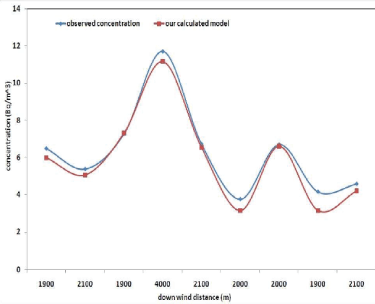Figure 1
Select your language of interest to view the total content in your interested language

### Article Usage

• Total views: 8570
• [From(publication date):
February-2016 - Aug 25, 2019]
• Breakdown by view type
• HTML page views : 8455
• PDF downloads : 115

## Post your commentCan't read the image? click here to refresh
###### Peer Reviewed Journals

Make the best use of Scientific Research and information from our 700 + peer reviewed, Open Access Journals

International Conferences 2019-20

Meet Inspiring Speakers and Experts at our 3000+ Global Annual Meetings

Top